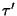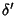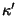## Klein's Equation

If a Real curve has no singularities except nodes and Cusps, Bitangents, and Inflection Points, thenwhereis the order,is the number of conjugate tangents,is the number of Real inflections,is the class,is the number of Real conjugate points, andis the number of Real Cusps. This is also called Klein's Theorem.